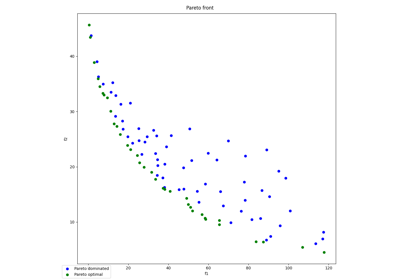gemseo / problems / analytical

# binh_korn module¶

Binh and Korn multi-objective problem.

This module implements the Binh and Korn multi-objective problem:

\begin{split}\begin{aligned} \text{minimize the objective function } & f_1(x, y) = 4x^2 + 4y^2 \\ & f_2(x, y) = (x-5)^2 + (y-5)^2 \\ \text{with respect to the design variables }&x,\,y \\ \text{subject to the general constraints } & g_1(x,y) = (x-5)^2 + y^2 \leq 25.0\\ & g_2(x, y) = (x-8)^2 + (y+3)^2 \geq 7.7\\ \text{subject to the bound constraints } & 0 \leq x \leq 5.0\\ & 0 \leq y \leq 3.0 \end{aligned}\end{split}
class gemseo.problems.analytical.binh_korn.BinhKorn(initial_values=(1.0, 1.0))[source]

Binh and Korn optimization problem.

The constructor initializes the BinhKorn OptimizationProblem by defining the DesignSpace, the objective function and the constraints.

Parameters:

initial_values (FigSizeType) –

Initial value of the design variables.

By default it is set to (1.0, 1.0).

constraints: list[MDOFunction]

The constraints.

current_iter: int

The current iteration.

database: Database

The database to store the optimization problem data.

design_space: DesignSpace

The design space on which the optimization problem is solved.

eq_tolerance: float

The tolerance for the equality constraints.

fd_step: float

The finite differences step.

ineq_tolerance: float

The tolerance for the inequality constraints.

max_iter: int

The maximum iteration.

minimize_objective: bool

Whether to maximize the objective.

new_iter_observables: list[MDOFunction]

The observables to be called at each new iterate.

nonproc_constraints: list[MDOFunction]

The non-processed constraints.

nonproc_new_iter_observables: list[MDOFunction]

The non-processed observables to be called at each new iterate.

nonproc_objective: MDOFunction

The non-processed objective function.

nonproc_observables: list[MDOFunction]

The non-processed observables.

observables: list[MDOFunction]

The observables.

pb_type: str

The type of optimization problem.

preprocess_options: dict

The options to pre-process the functions.

solution: OptimizationResult

The solution of the optimization problem.

stop_if_nan: bool

Whether the optimization stops when a function returns NaN.

use_standardized_objective: bool

Whether to use standardized objective for logging and post-processing.

The standardized objective corresponds to the original one expressed as a cost function to minimize. A DriverLibrary works with this standardized objective and the Database stores its values. However, for convenience, it may be more relevant to log the expression and the values of the original objective.

## Examples using BinhKorn¶Pareto front on Binh and Korn problem

Pareto front on Binh and Korn problem